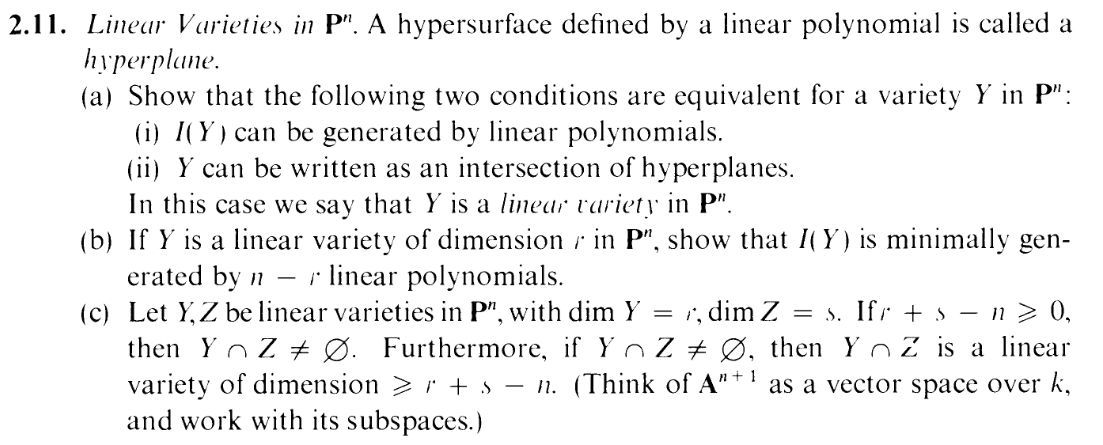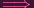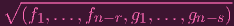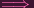I.2.11b&c

2/21/2021A little late on this one. Lazy weekend, complete with a super mega loli fap, but the call of accountability has brought me to update this blog. No worries: These two parts are meant to coast on part (a). And coast we shall. This is the relieving horizon after the technical nitty gritty. In fact, we'll use this as the theme song for today's post, reminding ourselves that even coasting still requires you to do things... Blame it on the cunny.

In fact, we basically kinda already did part (b). Here's a lemma to represent my hard work last time:

LEMMA: Gimme an I(Y ) generated by linear polynomials, say, g0,,gl. What I showed is that, we can actually generate I(Y ) with a set of s linear polys, f0,,fs-1 such that k[x0,xn]∕I(Y ) = k[xs,,xn].

So in our case, this implies:

 dim k[x0,…xn]∕I(Y ) = dim k[xs,…,xn]dim S(Y ) = n - s + 1dim Y + 1 = n - s + 1 ((2.6))dim Y = n - sr = n - ss = n - r

And remember, s is the cardinality of our generating set, so we can generate I(Y ) with n - r linear polynomials. And is it a minimal generating set? Duh. If we had a smaller generating set (which we can assume is comprised of linear elements without loss of generality because... you know.... think about it.... EXERCISE LEFT TO THE READER KEK), then applying the LEMMA would tell us that k[x0,,xn]∕I(Y ) is isomorphic to different polynomial ring with a smaller s (more indeterminates), which is impossible.

I should mention that the converse of (b) is also clearly true, thanks again to the LEMMA. So you can think of it as an if and only if.

Part (c) is easy...ish: Using part (b), use minimal generators I(Y ) = (f1,,fn-r), I(Z) = (g1,gn-s). So

 I(Y ∩ Z) = I(Z(f1,…,fn-r) ∩ Z(g1,…,gn-s)) (bolding Z for zero set to avoid ambiguity with the other Z lol) = I(Z(f1,…,fn-r,g1,…,gn-s)) == (f1,…,fn-r,g1,…,gn-s) (thanx to part (a))

So Y R is a linear variety (I mean... probably. It's still possible that it's empty. But I'll show shortly that's not the case). And how many polynomials is that?
 (n - r) + (n - s) = 2n - r - s = 2n - (r + s)

So a minimal generating set of linear polynomials for I(Y R) has cardinality l 2n - (r + s). Note:
 l ≤ 2n - (r + s) = n + (n - (r + s)) = n - (r + s - n) ≤ n - 0 = n
So we have n polynomials. And how many variables do we have? n + 1. Which means that the RREF of part (a) will have at least one column of free variables. I.e. the system representing Y Z will have a nontrivial solution, thus making it a nonempty linear variety
Also l 2n - (r + s)n - l r + s - n. Part (b) tells us that dim Y Z = n - l, so we actually have dim Y Z (r + s) - n DUN.

Some sidenotes: Thanks to the assumption that r + s - n 0, we can write dim Y Z 0. This confused me at first, because I associated dimension 0 with "empty set", so I thought I ran into a problem, but then I realized the "dimension 0" can actually just mean "singleton" (think P0), among other things.

Also back in part (a), I required in my work that s n + 1. The case s = n + 1 is actually an empty set, and this exercise reminds me that an empty set does NOT count as a variety (empty sets don't count as irreducible). So I should really have asserted that s < n + 1 instead.

And thus, this exercise has been COASTED.